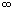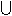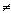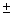SEARCH HOMEMath Central Quandaries & QueriesQuestion from Gale, a student: what does the term interval of the domain means?Gale, we have two responses:

Gale,

If you go to the Quandaries and Queries page and search for the term domain in the Quick Search window you will find a number of questions and answers concerning the domain of a function. I am not sure of the meaning of "interval of the domain". Can you send us the complete sentence where you saw this term?

Penny

Hi Gale.

The domain of a function is the set of all values that the function can take as an argument. For example, if f(x) = 3/x , then x can be any value other than 0 (because 3/0 is undefined).

An interval is a formal way of describing a continuous set of real values, and you can use it to describe a domain. In the example I gave, the interval would be (-, 0)(0, +). This corresponds to the more familiar notation x0.

Intervals are usually given as the union (hence thesymbol) of spans of possible values (which can be considered sub-intervals). The sub-interval (-, 0) means the set of all real numbers between, but not including negative infinity and 0. Round parentheses indicate that the endpoint of the range is not included and square brackets indicate that it is. For example, the interval corresponding to the inequality -1 ≤ b < 4 would be [-1, 4). The interval corresponding to the function f(x) = x would be (0, +) . Infinite endpoints aren't real numbers, so they always have round parentheses.

The quadratic y2 = x + 2 corresponds to y =(x + 2)½, so you can see immediately that x must be greater than or equal to negative two (otherwise x would be the square root of a negative number). So the interval of the domain here is  [-2, +).

Stephen La Rocque.>Math Central is supported by the University of Regina and The Pacific Institute for the Mathematical Sciences.